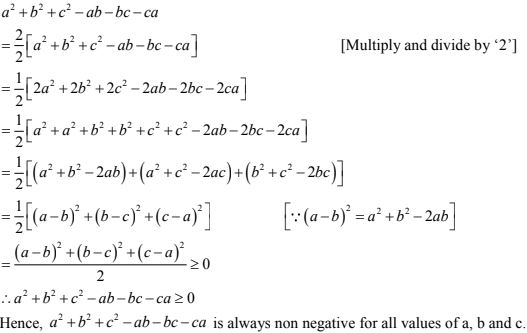# Chapter 4 Algebraic Identities RD Sharma Solutions Exercise 4.1 Class 9 Maths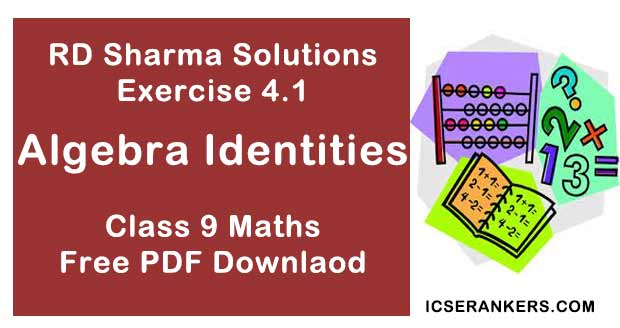Chapter Name RD Sharma Chapter 4 Algebraic Identities Exercise 4.1 Book Name RD Sharma Mathematics for Class 10 Other Exercises Exercise 4.2 Related Study NCERT Solutions for Class 10 Maths

### Exercise 4.1 Solutions

1. Evaluate each of the following using identities :
(i) (2x – 1/x)2
(ii) (2x + y)(2x – y)
(iii) (a2b – ab2)2
(iv) (a – 0.1)(a + 0.1)
(v) (1.5x2 – 0.3y2)(1.5x2 + 0.3y2)

Solution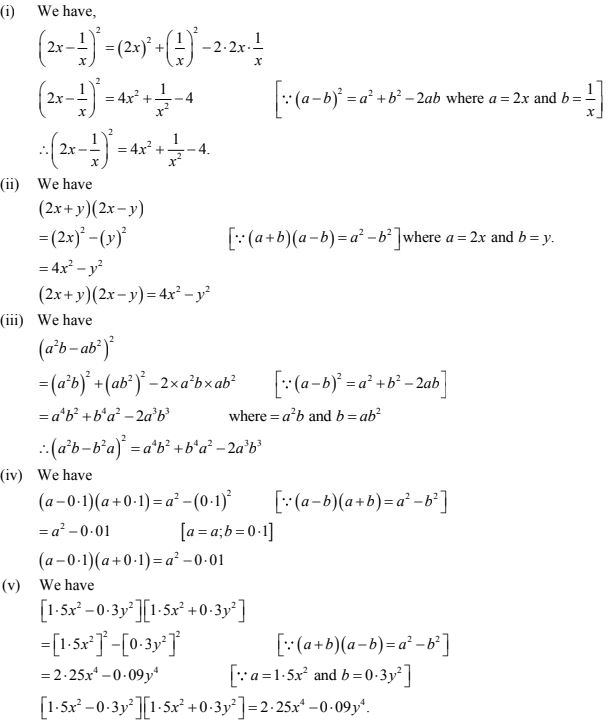2. Evaluate each of the following using identities :
(i) (399)2
(ii) (0.98)2
(iii) 991 × 1009
(iv) 117 × 83

Solution

(i) We have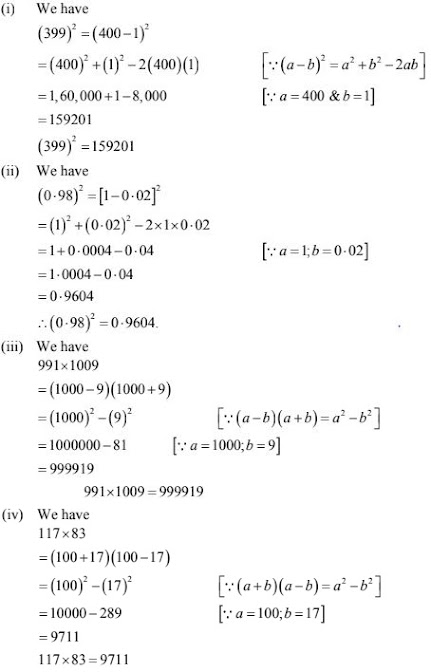3. Simplify each of the following :
(i) 175 × 175 + 2×175 ×25 + 25 ×25
(ii) 322 × 322 - 2 ×322 ×22 + 22 × 22
(iii) 0.76 ×0.76 + 2×0.76 ×0.24 + 0.24 ×0.24
(iv) (7.83 + 7.83 - 1.17 × 1.17)/6.66
Solution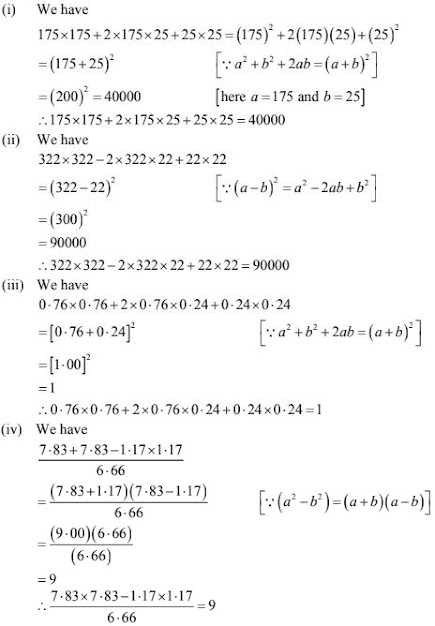5. If x - 1/x = -1, find the value of x2 + 1/x2 .
Solution
We have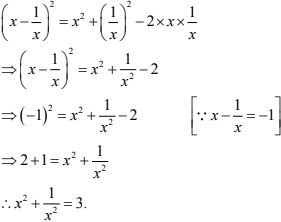6. If x + 1/x = √5, find the values of x2 + 1/x2 and x4 + 1/x4 .
Solution
We have,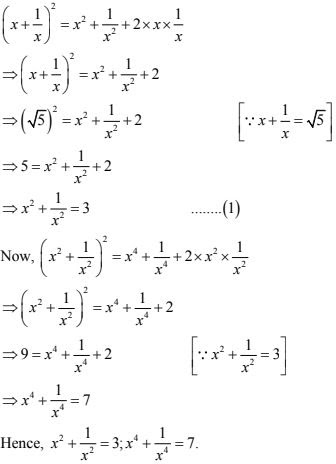7. If  x2 + 1/x2  = 66, find the value of x - 1/x
Solution
We have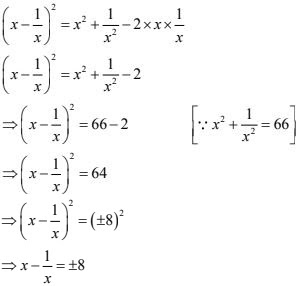8. If  x2 + 1/x2  = 79, find the value of x - 1/x.
Solution
We have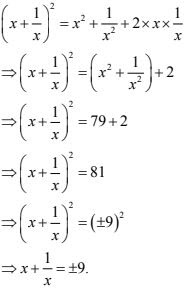9. If 9x2 + 25y2 = 181 and xy = -6, find the value of 3x + 5y
Solution
We have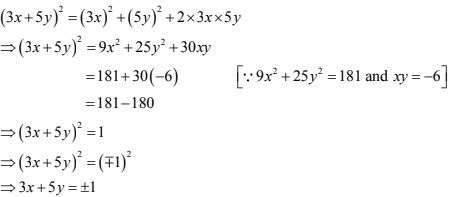10. If 2x + 3y = 8 and xy = 2, find the value of 4x2 + 9y2 .
Solution
We have,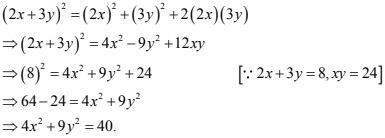11. If 3x -7y = 10 and xy = -1, find the value of 9x2 + 49y2 .
Solution
We have,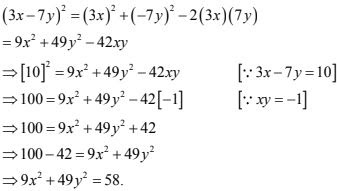12.Simplify each of the following products:
(i) (1/2 a - 3b)(1/2 a + 3b)(1/4 a2 + 9b2 )
(ii) (m +n/7)3 (m -n/7)
(iii) (x/2 - 2/5)(2/5 - x/2) - x2 + 2x
(iv) (x2 + x - 2)(x2 - x + 2)
(v) (x3 - 3x2 - x)(x2 - 3x + 1)
(vi) 2x4 - 4x2 + 1][2x4 - 4x2 - 1]
Solution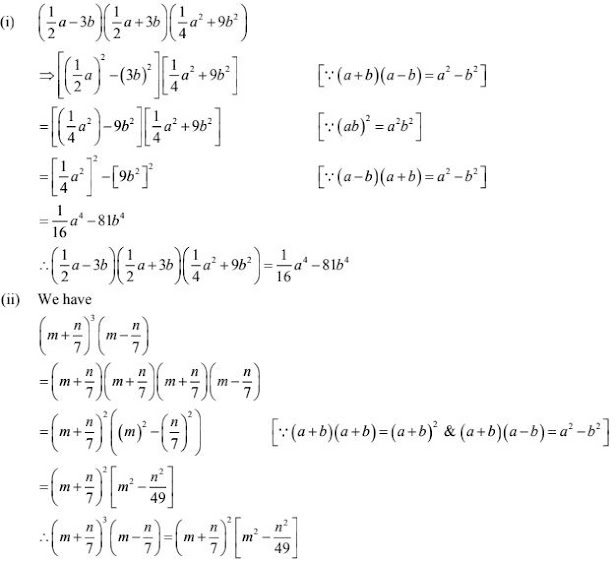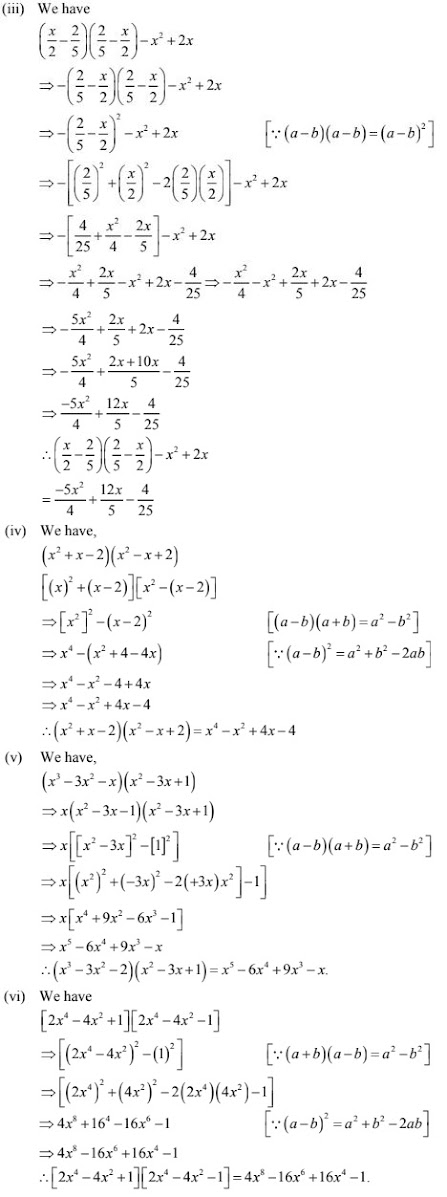13. Prove that a2 + b2 + c2 - ab - bc - ca is always non - negative for all values of a, b and c
Solution
We have